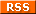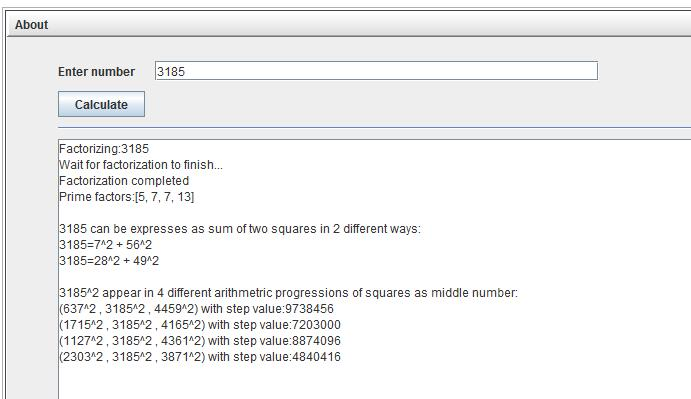# Squares in Arithmetic Progression

I have a passion for recreational mathematical puzzles. Especially combinatorical puzzles or puzzles involving square numbers. My approach is normally some mathematical research combined with brute force CPU power. Often I am not able to solve the puzzle, but sometimes I am. This is the sixth adventure in my series of 'mathematical adventures' and is part 2 of a 3 part adventure about AP of squares. Part I was an applet calculating AP of squares. In this part II, I will go into every detail and explain how the applet does this so fast. Finding squares in AP is fully solved mathematically and will reveal some less known facts about square numbers. While finding AP of squares is easy though tedious, there are still unsolved problems about some properties and this is what part III will be about.

## Abstract:

### First we have to start with the defintion of an Arithmetic Progression (AP). Definition of Arithmetic Progression (AP): An Arithmetic Progression is a sequence of integers such that the difference between the consecutive terms is constant. For instance the sequence: 9,13,17,21,25. (difference is 4) or the sequence: 1,25,49 (difference is 24) However I am only interested in AP where each integer in the sequence is a square! The first example of such an AP is 1,25,49 since these are squares: 12, 52, 72. (difference is 24). The first AP of squares (in terms of the middle number) can be seen in the picture to the right. So what is all the fuss about AP of squares? Well, they are the fundamental building blocks when trying to solve the holy grail of recreational mathematics, which is finding a magic square of squares of order 3. I have already touched this subject in Constructing magic square of squares.### No Four Squares In Arithmetic Progression:

In the examples of squares in AP I gave above, all included only 3 squares. The reason for this is that there does not exist an AP of squares of length 4 or higher. You can only find 3 squares in an AP. This was proven recently by Lee Morgenstern . It is no coincidence that it was proven by Lee Morgenstern since he has also been solving problems involving squares and Magic Square of Squares for years and is probably the one that knows most about this problem. Because of this result I will also refer to an AP of squares as a triplet and denote this by ( a2, b2, c2). So the problem is reduced to finding all AP of 3 squares!

### Fermat's theorem on sums of two squares:

This simple theorem is almost responsible for the whole story about finding the AP of squares.

A prime number p can be written as a sum of two square numbers if and only if p ≡ 1 (mod 4)
That is: p prime and p = x² + y² <=> p ≡ 1 (mod 4)

For example 5= 1²+2², while it is not possible to express 7 as a sum of two squares.The next step is asking the same question for composite numbers. The story is almost the same. Let p = (a²+b²) and q=(c²+d²). Then it can be checked pq=(ac-bd)²+(ad+bc)²=(ac+bd)²+(ad-bc)², that is the product can also be expressed as sum of two squares and in fact in two different ways. So it follows that numbers which only have prime factors that are ≡ 1 (mod 4) can also be written as sum of two squares, by doing this trick recursive. Also given p = (a²+b²) then pk²=((ak)²+(bk)²), so this covers prime factors which are ≡ 3 (mod 4) that appear an even number of times. The only case not covered now is if there is one (or more) primefactor ≡ 3 (mod 4) with odd multiplicity, and in this case the number can not be written as a sum of two squares. Also the other way is true as well. The following theorem sums this up.

### Sum of two squares theorem:

A positive integer n is the sum of two squares if and only if each prime factor p of n such that p ≡ 3 (mod 4) occurs to an even power in the prime factorization of n.
That is given n=2r * p1n1 * p2n2...pini*q1m1*q2m2...qjmj where all p's are ≡1 (mod 4) and all q's are ≡3 (mod 4), then m1,m2,...,mj must all be even.

Futhermore, if it can be written as sum of two squares, then the number of different decompositions can be calculated as:

#different decompositions of n in sum of two squares: d(n) = 0.5 * (n1+1)*(n2+1)...(ni+1)     (rounded down)

Proof of the two theorems above.
Interesting consequence: If you add two square numbers, the result will either be a prime p ≡ 1 (mod 4) or all prime factors will be p ≡ 1 (mod 4). Guess you did not know that!

### Creating an AP of squares from a decomposition of a square into a sum of two integers:

A square number can of course always be written as a sum of two squares n²=n²+0². But it can be written non-trivial if it has at least primefactor p ≡ 1 (mod 4). This follows from the 'Sum of two squares theorem'.

There is a 1-1 relation between a non-trivial decomposition of n²=r²+s² and an AP ( (r-s)², n² ,(r+s)² ).

If n²=r²+s², 1<=s<=r. Then (r-s)², n² , (r+s)² forms an AP progression of squares with step-value 2*r*s.
This follows easy from calculating n²-(r-s)² and (r+s)²-n².

And from and AP of squares (x²,n²,y²) you can also easy express n²=r²+s², by finding r and s using the step-value and substraction/addition equations.

### How to decompose a prime p ≡ 1 (mod 4) into a sum of two squares

The intelligent reader will now realize the only thing missing is how to decompose a number into a sum of two squares. The theorem above only speak of existance of such two numbers, not how these two numbers are found. Surprisingly this is done by using Euclid greatest common divisor algorithm(gcd) over gaussian integers (Z[i]). Once again complex numbers help us solve a problem involving natural numbers.
Given a prime p ≡ 1 (mod 4).
1) Find integer a>=2 such a((p-1)/2) ≡ -1 (mod p).
Simply try starting from a=2,3,4,... Every attempt has 50% chance for success as a((p-1)/2) will be ≡ 1 or -1 (mod p).
So this will only require a few attemps.
2) Let t ≡ a((p-1)/4) (mod p). That is t²+1 ≡ 0 (mod p)
We now have p | (t+i)(t-i) in Z[i]. And gcd(p,t+i) is not 1 or p. So gcd(p,t+i) is an irreducible factor of p in Z[i]
3) Calculate gcd(p,t+i) in Z[i].
4) If gcd(p,t+i)= x+iy, then p = x²+ y²

Example:
Lets try to decompose 29 into a sum of two squares.
1) Test a=2: a^((29-1)/2) ≡ -1 mod 29. This is true. So a=2 works.
2) 12 ≡ 2^7 mod 29. We now have 144+1 ≡ -0 mod 29. So 29|(12-i)(12+i) in Z[i]
3) Find gcd(29,12+i) in Z[i]
a1=29
a2=12+i
a1/a2= 29/(12+i)= 2+0i. (Actually 2.4+0.2i, but we are in Z[i], round to nearest integer).
reminder= 29-2*(12+i)= 29-24-2i=5-2i
a3 =5-2i
a2/a3= 12+i/5-2i = 2 + i
reminder (12+i)-(5-2i)(2+i)=12+i-10-5i+4i-2=0
a4=0 (we are done)
We have now found : (12+i)=(5-2i)(2+i) with (5-2i) highest common divisor.
That is gcd(29,12+i)= 5-2i.
4)We are done: 29=5²+ 2².
Of course you could have done this for 29 in your head much faster, but for very large numbers this is the fastest known way to do it.

### Algorithm for finding all AP of squares:

This is the complete algorithmm for finding all AP of squares with n² as middle number. That is all AP (x²,n²,y²).
1) Factorize n. (then you also know the factorisation for n²)
Given n=2r * p1n1 * p2n2...pini*q1m1*q2m2...qjmj where all p's are ≡ 1 (mod 4) and all q's are ≡ 3 (mod 4)

If there are no primefactors p ≡ 1 (mod 4) , then we are finished. There are no AP with n² as middle number.
If there exist a factor q ≡ 3 (mod 4) of odd multiplicity, then we are also finished. There are no AP with n² as middle number.

Rewrite n by multipling together all primefactors q ≡ 3 (mod 4)
That is:
n=2r*k*p1n1*p2n2...pjni, where k=q1m1 * q2m2...qimj

2) For all primefactors p ≡ 1 (mod 4) decompose p into a sum of two squares.
if r is even: decompose 2r = 2(r/2)2+02
if r is odd: decompose 2r = 2((r-1)/2)2+ 2((r-1)/2)2

3) We now have n written as a constant k and factors of the type (x²+y²).
n=k*(x12+y12)*(x22+y22)*...*(xk2+yk2) Remember to repeat the entries for the decomposition of p as many times as the multiplicy of p.

Next we square n.
n²=k²*(x12+y12)*(x22+y22)*...*(x2k2+y2k2)

4) Next use this identity recursive
(a²+b²)(c²+d²) =(ac-bd)²+(ad+bc)²=(ac+bd)²+(ad-bc)² (two different ways to rewrite it, but they can be identical though)
Start with the product of the first two factors of n² decomposed into a sum of two squares. Use the identity to rewrite this into single decomposion. Create a set having these two different decompositions. Next take the third decomposition factor from n² and once again multiply this into each element of the set using the identity. The new set will now have up to 4 elements each of the form (s² + t²). Repeat for all decomposed factors of n².

Now we have a set where all elements are decompositions of the form (s² + t²). And for each element we have:
n²=k²*(s² + t²)=(ks)² + (kt)², which is a decomposition of n² into the sum of two squares.

Note that in case r is even, it just be multiplied into the constant k. But I wanted to explain it simple.

5) For all the decompositions from 4) n²= (kr)² + (ks)² , r>=s,
Create the AP:
( (kr-ks)²,n²,(kr+ks)²) which have step-value 2*k²*r*s

However if s=0, that is n²= (kr)², we have to discard the AP this would generate as the step-value would be zero.

Congratulations! You can now find all AP of squares having a given square n² in the middle.
The time complexity of this algorithm for big numbers with unknown factorization is the factorization step 1).

Example:
Lets try finding all AP having 3185² as middle number.
1) 3185=7²*5*13,
We see from the factorization that AP does exist since all p ≡ 3 (mod 4) has even multiplicity. (the prime 7 has multiplicity 2)
3185= k*5*13, k=7².

2)We calculate (just guessing, not using the GCD method as we can solve this easy).
5=2²+1²
13=3²+2²

3) n²=k²*(2²+1²)*(2²+1²)*(3²+2²)*(3²+2²), k²=49²

4) Just as step 2) this step is very tedius to do by hand!
Now continue through the factors one at a time
(3²+2²)*{(0²+5²), (3²+4²)} -> {(1²+18²), (6²+17²), (10²+15²)}
(3²+2²)*{(1²+18²), (6²+17²), (10²+15²)} -> {(0²+65²), (16²+63²), (25²+60²),(33²+56²), (39²+52²)}

5)We discard the (0²+65²) solution. Using tbe other 4 gives the following 4 AP:
3185²=49²*(16²+63²) => AP (49²(63-16)², 3185² , 49²(16+63)²) => AP (2303², 3185² , 3871²)
3185²=49²*(25²+60²) => AP (49²(60-25)² , 3185² , 49²(60+25)²) => AP (1715², 3185² , 4165²)
3185²=49²*(33²+56²) => AP (49²(56-33)² , 3185² , 49²(56+33)²) => AP (1127², 3185² , 4361²)
3185²=49²*(39²+52²) => AP (49²(52-39)² , 3185² , 49²(52+39)²) => AP (637², 3185² , 4459²)
And we are done!

### Using my applet to calculate AP of squares:

Below is a screenshot where I have typed the same problem into the applet. The solution is identical to the one we just calculated of course. Click the image to open the applet and play with it yourself.### Contact information:

If you have any questions or contributions to the problem you can contact me at thomas.egense@gmail.com